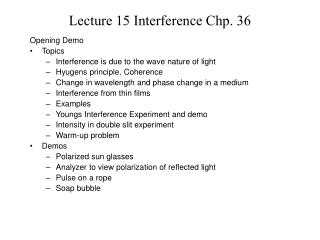Download PresentationLecture 15 Interference Chp. 36

# Lecture 15 Interference Chp. 36 - PowerPoint PPT Presentation

Lecture 15 Interference Chp. 36. Opening Demo Topics Interference is due to the wave nature of light Hyugens principle, Coherence Change in wavelength and phase change in a medium Interference from thin films Examples Youngs Interference Experiment and demoI am the owner, or an agent authorized to act on behalf of the owner, of the copyrighted work described.
Download Presentation## Lecture 15 Interference Chp. 36

Download Policy: Content on the Website is provided to you AS IS for your information and personal use and may not be sold / licensed / shared on other websites without getting consent from its author.While downloading, if for some reason you are not able to download a presentation, the publisher may have deleted the file from their server.

- - - - - - - - - - - - - - - - - - - - - - - - - - E N D - - - - - - - - - - - - - - - - - - - - - - - - - -
Presentation Transcript
1. Lecture 15 Interference Chp. 36 Opening Demo • Topics • Interference is due to the wave nature of light • Hyugens principle, Coherence • Change in wavelength and phase change in a medium • Interference from thin films • Examples • Youngs Interference Experiment and demo • Intensity in double slit experiment • Warm-up problem • Demos • Polarized sun glasses • Analyzer to view polarization of reflected light • Pulse on a rope • Soap bubble

2. Huygen’s Principle, Wavefronts and Coherence Examples of coherence are: Laser light Small spot on tungsten filament Wavefront k Most light is incoherent: Two separate lightbulbs Two headlight beams on a car Sun is basically incoherent

3. Three ways to get light out of phase 1. Rays go through different material with different index of refraction 2. Reflection from a medium with greater index of refraction 3. The selected rays travel different distances. Now lets look at examples

4. Vacuum Vacuum c = f l n=c/v vn= f ln =f l/n Concept of path length difference, phase and index of refraction N2=L/ ln2=Ln2/ l N1=L/ ln1 N2 - N1=L(n2-n1)/l Path length difference = Phase difference = L(n2-n1)/l Rays are in phase if L(n2-n1)/l =m l m=1, 2, 3.. Rays are out of phase if L(n2-n1)/l =(m+1/2) l m=0, 1, 2, 3.. 1 l is the same as 2p rad,l/2 is the same as p rad, etc.

5. Out of Phase by 180 degrees or p radians or l/2 In between In Phase

6. air 1.0 Reflection eye 1 2 soap 1.30 L air 1.00 180 deg phase change Thin film Interference Phenomenon: Reflection First consider phase change upon reflection Now consider the path length differences Constructive Interference

7. 2 1 Thin film Interference PhenomenonTransmission air 1.0 Transmission Constructive interference soap 1.30 L air 1.00 m = 1,2,3,4…. n =1.30 eye No phase changes upon reflection

8. 39. A disabled tanker leaks kerosene (n = 1.20) into the Persian Gulf, creating a large slick on top of the water (n = 1.30). (a) If you are looking straight down from an airplane while the Sun is overhead at a region of the slick where its thickness is L=460 nm, for which wavelength(s) of visible light is the reflection brightest because of constructive interference? air 1.0 1 2 Kerosene 1.20 L Water 1.30 180 deg phase change Path difference between ray 1and ray 2 = 2L. Phase changes cancel out For constructive interference path difference must = integral number of wavelengths We note that only the 552 nm wavelength falls within the visible light range.

9. air 1.0 Kerosene 1.20 Water 1.30 L 2 1 (b) If you are scuba diving directly under this same region of the slick, for which wavelength(s) of visible light is the transmitted intensity strongest? (Hint: use figure (a) with appropriate indices of refraction.) For transmission, ray 2 undergoes 180 deg phase shift upon reflection at the Kerosene-water interface. Therefore, for constructive interference 2L= integral number of wavelengths in n2 plushalf a wavelength. Scuba diver

10. Visible spectrum is 430 nm - 690 nm We note that only the 441.6 nm wavelength (blue) is in the visible range,

11. x The wave from S1 travels a distance x and the wave from S2 travels a distance The path difference is detector 27. S1 and S2 in Fig. 36-29 are point sources of electromagnetic waves of wavelength 1.00 m. They are in phase and separated by d = 4.00 m, and they emit at the same power. (a) If a detector is moved to the right along the x-axis from source S1, at what distances from S1 are the first three interference maxima detected?

12. x For constructive interference we have The solution for x of this equation is

13. Solve for x

14. m=3 m=2 m=1 What about m = 4 ? This corresponds to x=0. Path difference =4 meters.

15. Where do the minima occur? m=3 m=2 m=1 m=1 m=0 m=0 x=15.75 m m=1 x =4.55 m m=2 x=1.95 m m=3 x= 0.55 m Although the amplitudes are the same at the sources, the waves travel different distances to get to the points of minimum intensity and each amplitude decreases in inverse proportion to the square of the distance traveled. The intensity is not zero at the minima positions.

16. Young’s Double SlitInterference Experiment m=2 y m=1 q m=0 m=1 m=2 D